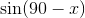Trigonometry : Complementary and Supplementary Identities

Example Questions

Example Question #1 : Complementary And Supplementary Identities

Given that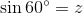, which of the following must also be true?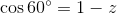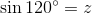None of these must also be true.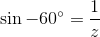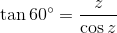Explanation:

The supplementary angle identity states that in the positive quadrants, if two angles are supplementary (add to 180 degrees), they must have the same sine. In other words,

if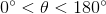, then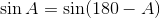Using this, we can see that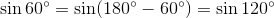Thus, if, thenalso.

Example Question #2 : Complementary And Supplementary Identities

Which of the following is equivalent to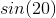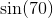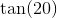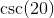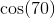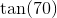Explanation:

Recall that the cosine and sine of complementary angles are equal.

Thus, we are looking for the complement of 70, which gives us 20.

When we take the cosine of this, we will get an equivalent statement.

Example Question #3 : Complementary And Supplementary Identities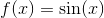Which of the following is equivalent to the function above.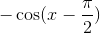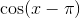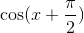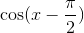Explanation:

The cosine graph at zero has a peak at 1.

The first peak in the sine curve is at π/2, so you adjust the cosine with a -π/2 and that gives the answerExample Question #4 : Complementary And Supplementary Identities

Simplify the following expression: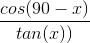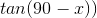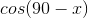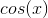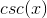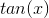Explanation:

Simplifying this expression relies on an understanding of the cofunction identities.  The cofunction identities hinge on the fact that the value of a trig function of a particular angle is equal to the value of the cofunction of the the complement of that angle.  In other words,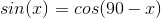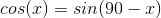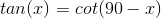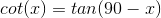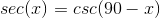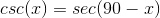That means that returning to our initial expression, we can do some substiution.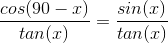We then turn to another identity, namely the fact that tangent is just the quotient of sine and cosine.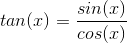We can substitute again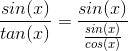Yet dividing by a fraction is the same as multipying by the reciprocal.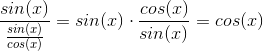With some cancellation, we have arrived at our answer.

Example Question #1 : Complementary And Supplementary Identities

Which of those below is equivalent to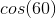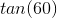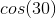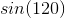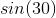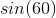Explanation:

Think of a right triangle. The two non-right angles are complementary since the angles in a triangle add up to 180. As a result, when we apply the definitions of sine and cosine, we get that the sine of one of these angles is equivalent to the cosine of the other. As a result, the sine of one angle is equal to the cosine of its complement and vice versa.

Therefore, when we look at the angles of a right triangle that includes a 60 degree angle we get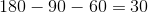.

Thus the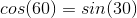Example Question #6 : Complementary And Supplementary Identities

Which one is equal to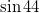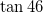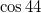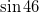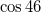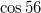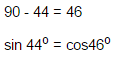Complementary angles are equal to one's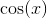to others